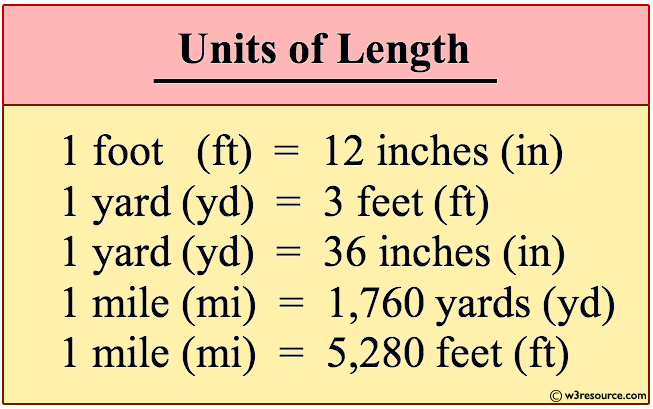﻿ Python: Convert the distance (in feet) to inches, yards, and miles - w3resource# Python: Convert the distance (in feet) to inches, yards, and miles

## Python Basic: Exercise-61 with Solution

Write a Python program to convert the distance (in feet) to inches, yards, and miles.

Unit Equivalents Conversion Factors
(longer to shorter units of measurement)
Conversion Factors
(shorter to longer units of measurement)
1 foot = 12 inches 12 inches
_______
1 foot
1 foot
_______
12 inches
1 yard = 3 feet 3 feet
_______
1 yard
1 yard
_______
3 feet
1 mile = 5,280 feet 5,280 feet
________
1 mile
1 mile
________
5,280 feet

Pictorial Presentation:Sample Solution:

Python Code :

``````d_ft = int(input("Input distance in feet: "))
d_inches = d_ft * 12
d_yards = d_ft / 3.0
d_miles = d_ft / 5280.0

print("The distance in inches is %i inches." % d_inches)
print("The distance in yards is %.2f yards." % d_yards)
print("The distance in miles is %.2f miles." % d_miles)
```
```

Sample Output:

```Input distance in feet: 100
The distance in inches is 1200 inches.
The distance in yards is 33.33 yards.
The distance in miles is 0.02 miles.
```

## Visualize Python code execution:

The following tool visualize what the computer is doing step-by-step as it executes the said program:

Python Code Editor:

Have another way to solve this solution? Contribute your code (and comments) through Disqus.

What is the difficulty level of this exercise?

Test your Python skills with w3resource's quiz

﻿

## Python: Tips of the Day

List comprehension:

```>>> m = [x ** 2 for x in range(5)]
>>> m
[0, 1, 4, 9, 16]
```